# Compound Interest Questions for Competitive Exams

Compound Interest Questions with solution for Competitive Exams. Important and repeated questions from the previous year SSC CGL, CHSL, CPO, JE and GD Exams. Very useful for upcoming NRA CET, UPSSSC UP PET, IBPS Bank PO and Clerks examinations.

## Formula of Compound Interest

Compound Interest =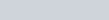$P (1+\frac{r}{n})^{nt} - P$
Total Accumulated Amount =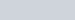$P(1+\frac{r}{n})^{nt}$
P= Principal Amount
r = Rate of Interest
n = compound frequency per year
t = time (in year)

## Compound Interest Questions for Competitive Exams

Q.1: The compound interest on ₹ 12000 for 9 months at 20% per annum, interest being compounded quarterly is :
a) ₹ 1750
b) ₹ 2089.70
c) ₹ 1891.50
d) ₹ 2136.40

Ans : c) ₹ 1891.50
Rate of Interest => Yearly = 20, Quarterly = 20/4 = 5%
Time => 9 months = 3 quarter
Compound Interest =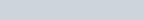$12000[(1 + \frac {5}{100})^3 - 1]$
=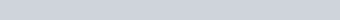$12000[(\frac {21}{20})^3 -1] = 12000 \times \frac{21}{20} \times \frac{21}{20} \times \frac{21}{20} -12000$ =₹ 1891.5

Q.2: In what time will ₹ 64000 amount to ₹ 68,921 at 5% per annum, interest being compounded half yearly?
a) 3 years
b) 2 year 6 months
c) 2 years
d) 1 year 6 months

Ans : d) 1 year 6 months
Rate of Interest 2.5% per half year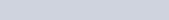$68921 = 64000 (1+\frac {5}{200})^t$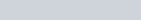$(\frac {41}{40})^t = \frac {68921}{64000} = (\frac {41}{40})^3$
=> t = 3 half years = 1 year 6 months

Q.3: The principal that yields a compound interest of ₹ 420 during the second year at 5% per annum is :
a) ₹ 7000
b) ₹ 5000
c) ₹ 8000
d) ₹ 6000

Ans : c) ₹ 8000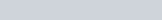$P(1+\frac {R}{100}) \times \frac {R}{100} = 420$$P(1+\frac {5}{100}) \times \frac {5}{100} = 420$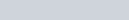$P =420 \times 20 \times \frac {20}{21}$ = ₹ 80000

Q.4: The compound interest on a sum of money for 2 years is ₹ 615 and the simple interest for the same period is ₹ 600. Find the principal.
a) ₹ 6500
b) ₹ 6000
c) ₹ 8000
d) ₹ 9500

Ans : b) ₹ 6000
Simple Interest for one year = 300
Interest on ₹ 300 = 615-600 = ₹ 15
Rate =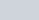$\frac {15 \times 100}{300\times 1}$ = 5%
Simple Interest =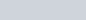$\frac {P \times 5 \times 2}{100} =600$
=> P = ₹ 6000

Q.5: ₹ 800 at 5% per annum compounded annually will amount to ₹ 882 in how many years:
a) 1 year
b) 2 year
c) 3 year
d) 4 year

Ans: b) 2 year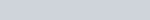$(1+\frac{5}{100})^t = \frac {882}{800} = \frac{441}{400}$
=>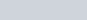$(\frac {21}{20})^t = (\frac {21}{20})^2$
=> t = 2 years

Q.6: A man saves ₹ 2000 at the end of each year and invests the money at 5% compound interest. At the end of 3 years he will have :
a) ₹ 4305
b) ₹ 6305
c) ₹ 4205
d) ₹ 2205

Ans : b) ₹ 6305
Amount =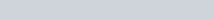$2000(1+\frac {5}{100})^2 + 2000(1+\frac {5}{100})$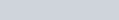$= 2000 \times \frac{21}{20}\times \frac{41}{20}$ = ₹ 4305
At the end of 3 year 4305 + 2000 (Saving of 3rd year = 6325

Q.7: If the rate of interest be 4% per annum for first year, 5% per annum for second year and 6% per annum for third year, then the compound interest of ₹ 10000 for 3 years will be :
a) ₹ 1600
b) ₹ 1625.80
c) ₹ 1575.20
d) ₹ 2000

Ans : c) ₹ 1575.20
A =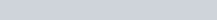$10000(1+\frac{4}{100})(1+\frac{5}{100})(1+\frac {6}{100}$
=$10000 \times \frac {26}{25} \times \frac {21}{20} \times \frac {53}{50}$ = ₹ 1575.20

Q.8: The compound interest on ₹ 8000 at 15% per annum for 2 years 4 months, when compounded annually is :
a) ₹ 2980
b) ₹ 3091
c) ₹ 3109
d) ₹ 3100

Ans : c) ₹ 3109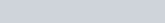$Amount = P\left ( 1+\frac{R}{100} \right )^{t}$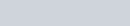$= 8000\left ( 1+\frac{15}{100} \right )^{2\frac{1}{3}}$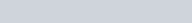$= 8000\left ( 1+\frac{3}{20} \right )^{2}\left ( 1+\frac{3}{20\times 3} \right )$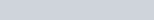$= 8000\times \frac{23}{20}\times\frac{23}{20}\times\frac{21}{20}$ = ₹ 11109
Compound Interest = 11109 – 8000 = ₹ 3109

## Based on Both Compound and Simple Interest

Q.9: A certain amount of money earns ₹ 540 as simple interest in 3 years. If it earns a compound interest of ₹ 376.20 at the same rate of interest in 2 years, find the amount (in ₹).
a) 1600
b) 1800
c) 2000
d) 2100

Ans : c) 2000
Solution:
3 Year Simple Interest = 549
1 year Simple Interest =₹180
2 year Simple Interest = 360
Compound Interest for 2 years =376.20
Difference = 16.20
₹16.20 is Interest on Simple Interest of First year (i.e. 180)
Rate =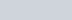$\frac {16.20}{180} \times 100$ = 9% per annum
Principal =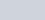$\frac {180 \times 100}{1\times9}$ =₹2000

Q.10: There is 100% increase to an amount in 8 years, at simple interest. Find the compound interest of ₹ 8000 after 2 years at the same rate of interest.
a) ₹ 2500
b) ₹ 2000
c) ₹ 2250
d) ₹ 2125

Ans : d) ₹ 2125
Solutions :
Rate =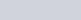$\frac{100\times100}{100\times8} = \frac {25}{2}$%
Compound Interest =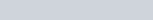$8000(1+\frac {25}{200})^2 - 8000$
=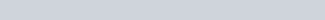$(8000 \times \frac98 \times \frac98) - 8000 =10125 -8000 =2125$

Q.11: The compound interest on a certain sum of money invested for 2 years at 5% per annum is ₹ 328. The simple interest on the sum, at the same rate and for the same period will be:
a) ₹ 320
b) ₹ 308
c) ₹ 300
d) ₹ 287

Ans : a) ₹ 320
Solution: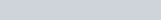$328 =P(1+\frac {5}{100})^2 -P$
=>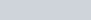$328 = P(\frac{41}{400})$
P = ₹3200
Simple Interest =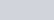$\frac{3200\times5\times2}{100}$ =₹320

Q.12: The compound interest on a certain sum for two successive years are 225 and ₹ 238.50. The rate of interest per annum is :
a) 7.5%
b) 5%
c) 10%
d) 6%

Ans: d) 6%
Solution: Simple Interest on ₹ 225 for 1 year = 238.50 – 225 = 13.50
Rate =$\frac {13.50 \times 100}{225 \times 1}$ = 6%

## Difference between Compound and Simple Interest

Q.13: The difference between the simple and compound interest on a certain sum of money for 2 years at 4% per annum is ₹ 4. The sum is :
a) ₹ 2500
b) ₹ 2400
c) ₹ 2600
d) ₹ 2000

Ans: a) ₹ 2500
t= 2 years, r = 4% per annum
Difference in CI and SI = ₹ 4 = Sum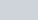$(\frac{4}{100})^2$
Sum = ₹ 2500

Q.14: The difference between compound and simple interest on a certain sum for 3 years at 5% per annum is ₹ 122. The sum is :
a) ₹ 16000
b) ₹ 15000
c) ₹ 12000
d) ₹ 10000

Ans : a) ₹ 16000
Solution :
Difference = Sum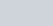$\frac {r^2(300+r) }{(100)^3}$
Sum =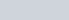$\frac {122\times 1000000}{25 \times 305}$ = ₹16000

Q.15: The difference between simple and compound interest (compounded annually) on a sum of money for 2 years at 10% per annum is ₹ 65. The sum is :
a) ₹ 65650
b) ₹ 6565
c) ₹ 65065
d) ₹ 6500

Ans: d) ₹ 6500
Solutions :
Difference for two years= Sum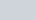$(\frac {r}{100})^t$
65 = Sum$(\frac {10}{100})^2$
Sum =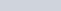$65 \times 100$ = ₹ 6500

## If the Amount Becomes n Times of the Sum After t Years at Compound Interest

Q.16: A sum of money placed at compound interest doubles itself in 5 years. In how many years, it would amount to eight times of itself at the same rate of interest ?
a) 10 years
b) 15 years
c) 7 years
d) 20 years

Ans: b) 15 years
2 times in 5 years
4 times in 10 years
8 times in 15 years

Q.17: A sum of money becomes 1.331 times in 3 years as compound interest. The rate of interest is:
a) 8%
b) 7.5%
c) 10%
d) 50%

Ans: c) 10%
Solutions :
Let Principal = 1000, Amount = 1331$1331 = 1000 ( 1+ \frac {r}{100})^3$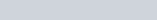$\frac {1331}{1000} = (\frac {11}{10})^3= ( \frac {100+r}{100})^3$
100 +r = 110, => r =10%

### Installments : Compound Interest Questions for Competitive Exams

Q.18: A sum of ₹ 210 was taken as a loan. This is to be paid back in two equal instalments. If the rate of interest be 10% compounded annually, then the value of each instalment is :
a) ₹ 127
b) ₹ 121
c) ₹ 210
d) ₹ 225

Ans : b) ₹ 121
Solution: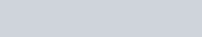$210 = \dfrac {x}{(1+\frac {10}{100})} + \dfrac {x}{(1+\frac {10}{100})^2}$
=>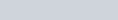$\frac {10}{11}x + \frac {100}{121}x = 210$
=>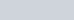$\frac {210}{121} x = 210$
=>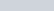$x = 121$

Q.19: A sum of money is paid back in two annual instalments of `17640 each, allowing 5% compound interest compounded annually. The sum borrowed was :
a) ₹ 32800
b) ₹ 32200
c) ₹ 32000
d) ₹ 32400

Sum Borrowed =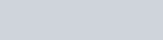$[\dfrac {17640}{(1+\frac {5}{100})} + \dfrac {17640}{(1+\frac{5}{100})^2}]$ =₹ 32800